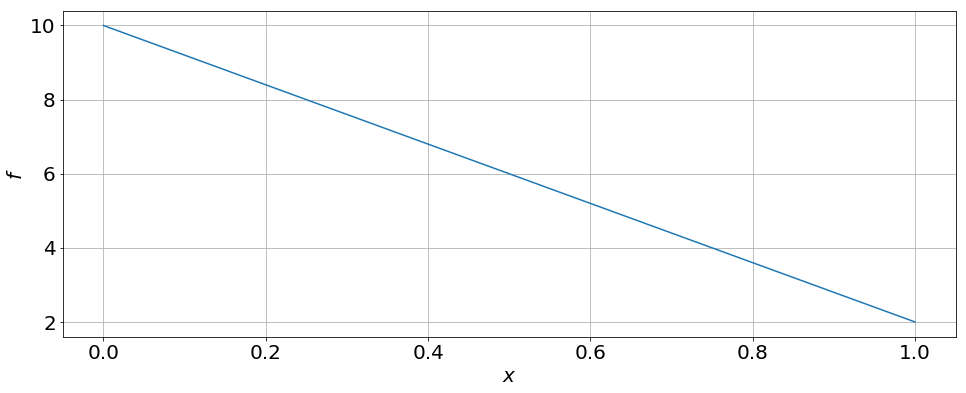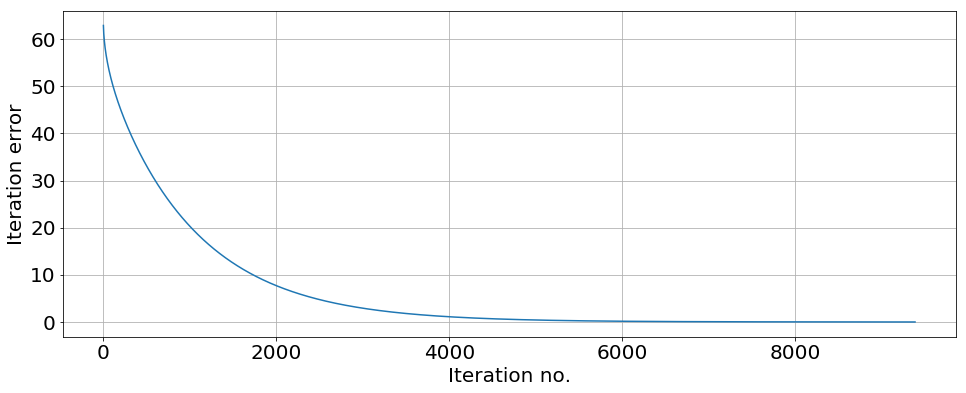# The Gauss-Seidel Method¶

### Modules - Partial Differential Equations¶

Last edited: February 7th 2018

In this module we will look at a method for solving a linear system of equations using a iterative method called the Gauss-Seidel method . The fact that it is an iterative method means that one has to iterate the solution algorithm many times in order to get an answer with an accuracy below a given tolerance. This is opposed to direct methods where one iteration of the algorithm gives the solution directly. However, the algorithm for a direct method can have certain limitations regarding where they can be applied, which make iterative methods useful in many situations.

If we have the linear system of equations $$A {\bf{x}} = {\bf{b}}$$ the Gauss-Seidel method is based on making the decomposition of $A$ in to a lower diagonal matrix $L$ and upper diagonal matrix $U$: \begin{align} A = \left(\matrix{ a_{11} & a_{12} & a_{13} & ...\\ a_{21} & a_{22} & a_{23} & ...\\ a_{31} & a_{32} & a_{33} & \ddots \\ \vdots & \vdots & \vdots & \ddots }\right) = \left(\matrix{ a_{11} & 0 & 0 & ...\\ a_{21} & a_{22} & 0 & ...\\ a_{31} & a_{32} & a_{33} & \ddots \\ \vdots & \vdots & \vdots & \ddots }\right) + \left(\matrix{ 0 & a_{12} & a_{13} & ...\\ 0 & 0 & a_{23} & ...\\ 0 & 0 & 0 & \ddots \\ \vdots & \vdots & \vdots & \ddots }\right) \equiv L + U\\ \phantom{+} \end{align} This can be used to get $\bf{x}$ at iteration $k+1$ by using the solution from the previous iteration: $$L{\bf{x}}^{k+1} = {\bf{b}} - U{\bf{x}}^{k}.$$ If we now look at the equation for each element in ${\bf{x}}$ we get \begin{align} a_{11}x^{k+1}_1 &= b_1 - \sum_{i=2} a_{1i}x^{k}_i \quad \Rightarrow \quad x^{k+1}_1 = \frac{b_1}{a_{11}} - \frac{1}{a_{11}}\sum_{i=2} a_{1i}x^{k}_i,\\ a_{21}x^{k+1}_1 + a_{22}x^{k+1}_2 &= b_2 - \sum_{i=3} a_{2i}x^{k}_i \quad \Rightarrow \quad x^{k+1}_2 = \frac{b_2}{a_{22}} - \frac{1}{a_{22}}\sum_{i=3} a_{2i}x^{k}_i - \frac{a_{21}}{a_{22}}x_1^{k+1}, \end{align} etc. Here we see that in the second line we use the value of $x_1^{k+1}$ found on the line above. From the above we can deduce the general algorithm, namely that the value of $x_i^{k+1}$ is found by $$x_i^{k+1} = \frac{b_i}{a_{ii}} - \frac{1}{a_{ii}}\sum_{j>i} a_{ij} x_j^{k} - \frac{1}{a_{ii}}\sum_{j<i} a_{ij}x_j^{k+1}.$$ Note: If in the last term we had $k$ instead of $k+1$, this would correspond to the Jacobi method. However, since we here use the already calculated new values wherever possible, the Gauss-Seidel method converges faster than the Jacobi method.

#### Initial Guess¶

In order to get a solution at iteration $k+1$, we use the solution at iteration $k$. But what is the solution at iteration $0$? This has to be set explicitly as an initial guess! A good guess means fewer iterations are needed in order to get an accurate solution.

#### Stopping Criterion¶

But how do we know when to stop the iteration? One option is to iterate a given number of times no matter what, and keep the solution this gives. This option has obvious flaws: First of all there is no way of knowing how good the obtained solution is. Secondly, it might be a huge waste of time to iterate 1000 times if the solution was good enough after e.g. 300 iterations.

The second option is to look at how much the solution changes from iteration to iteration, and stop iterating when the change is smaller than a given value. One way to do this is to set the stopping criterion as a tolerance times the 2-norm of the difference between the initial guess and the first iteration. The 2-norm of a vector ${\bf{x}}$ is given by $$||{\bf x}||_2 = \left(\sum_{i=1}^n \left|x_i\right|^2\right)^{1/2}.$$ Though this might be a better option than choosing a fixed number of iterations, it might still not be the perfect solution. If for instance the initial guess is a very bad one, the 2-norm of the difference after the first iteration might be very big, hence demaning the tolerance to be set very low in order to get good accuracy. This also means that the method has to converge, or else we will get an infinite number of iterations.

#### Convergence¶

The Gauss-Seidel method will converge when the matrix $A$ is diagonally dominant, i.e. if the following conditions hold: \begin{align} a_{ii} &\ge \sum_{j\ne i} |a_{ij}| \qquad \forall~i,\\ \exists~i:\qquad a_{ii} &> \sum_{j\ne i} |a_{ij}|.\end{align} If this does not hold, the method is not guaranteed to converge.

### Example: Laplace's Equation¶

As an example on how to use the Gauss-Seidel method, we will solve the Laplace equation in one dimension with Dirichlet boundary conditions on both sides, i.e. $$\frac{d^2}{dx^2} f = 0$$ with $f(0) = f_0 = 10$ and $f(1) = f_1 = 2$. We discretize the $x$-axis using N+1 grid points: $x_i = i\Delta_x$ where $\Delta x = 1/(N+1)$ and $i\in [0, N+1]$. Hence we want to find the solution for $f(x_i) = f_i$ for $i\in[1, N]$. We use second order central differences to discretize the second derivative, $$\frac{d^2}{dx^2} f(x_i)\quad\rightarrow\quad \frac{f_{i+1} - 2f_i + f_{i-1}}{\Delta x^2}.$$

Using this discretization we can write the Laplace equation as a linear system of equations $A{\bf{f}} = {\bf b}$, where \begin{align} A = \left(\matrix{ 2 & -1 & 0 & 0 & ...\\ -1 & 2 & -1 & 0 & ...\\ 0 & -1& 2& -1 & ...\\ \vdots & \vdots & \vdots & \vdots & \ddots }\right),\\ \phantom{+} \end{align} and the source of ${ \bf b}$ will be discussed below. Here we have multiplied both sides of the Laplace equation with $-\Delta x^2$. First we check if $A$ is diagonally dominant.

First of all we see that for all rows except the first and last we have $|a_{ii}| = |a_{i,i+1}| + |a_{i,i-1}| = 2$. Hence the first condition holds. We also see that for the first row $|a_{11}| = 2 > |a_{12}| = 1$. Hence the second condition also holds. Ergo, $A$ is diagonally dominant.

Next, special care has to be shown for the cases $i=1$ and $i=N$, since we then have to use the bondary conditions. For $i=1$ that case we get a constant $f_0$ on the right hand side. Similarily, we get for $i=N$, we get a constant $f_1$ on the right hand side. Hence the vectr ${\bf b}$ consists only of $f_0$ as the first element, and $f_1$ as the last, $N$-the element. All other elements are zero.

#### Exact solution¶

The exact solution of the Laplace equation with the given boundary conditions is easily obtainable. Integrating twice with respect to $x$ we get $$f(x) = Ax + B.$$ Using the first boundary condition, we get $B = f_0 = 10$. At the second boundary we then get $A+10 = 2$, i.e. $A = -8$. Hence the exact solution is $f(x) = -8x+10$.

Below we import the necessary libraries and determine ${\bf f}$ using the Gauss-Seidel method. In order to see how the solution converges towards the exact solution, we will plot the 2-norm of the difference between the exact solution and the solution at iteration $k$.

In :
import numpy as np
import matplotlib.pyplot as plt
from datetime import datetime as datetime
newparams = {
'figure.figsize': (16, 6), 'axes.grid': True,
'lines.linewidth': 1.5,
'font.size': 20
}
plt.rcParams.update(newparams)

In :
# Number of grid points
N = 100
dx = 1/(N+1)
x = np.linspace(dx, 1-dx, N)

# Exact solution
f_e = -8*x+10

# Tolerance
tol = 1e-6
diff = 1
it_error = []

# Difference first round
diff0 = 0

# Boundary conditions
f0 = 10
f1 = 2

# Diagonal elements
d = 2

# Off-diagonal elements
e = -1

# Intitialize matrix and set elements
A = np.zeros([N, N])
for i in range(N):
A[i, i] = d
if i < N-1:
A[i, i+1] = e
if i > 0:
A[i, i-1] = e

# Initialize b-vector
b = np.zeros(N)
b = f0
b[N-1] = f1

# Inititializing solution array, use zeros as initial guess
f = np.zeros(N)

n_iter = 0

start = datetime.now()

while diff > tol*diff0:
f_new = np.zeros(N)
for i in range(N):
if i == 0:
f_new[i] = (b[i] - A[i, i+1]*f[i+1])/A[i, i]
elif i == N-1:
f_new[i] = (b[i] - A[i, i-1]*f_new[i-1])/A[i, i]
else:
f_new[i] = (b[i] - A[i, i+1]*f[i+1] - A[i, i-1]*f_new[i-1])/A[i, i]

# Determine 2-norm of differnce between iterations
diff = np.sqrt(sum((f_new-f)**2))

# Set 2-norm of difference in first iteration
if diff0 == 0:
diff0 = diff

it_error.append(np.sqrt(sum((f_new-f_e)**2)))

# Update f
f = f_new

n_iter += 1

print("Total time spent on iteration: ", datetime.now() - start)

print("Total number of iterations: ", n_iter)

print("2-norm of difference between solution and exact solution: ", it_error[-1])

x = np.linspace(0, 1, N+2)
ftot = np.concatenate(([f0], f, [f1]))

plt.figure()
plt.plot(x, ftot)
plt.xlabel('$x$')
plt.ylabel('$f$')

plt.figure()
plt.plot(it_error)
plt.xlabel('Iteration no.')
plt.ylabel('Iteration error')
plt.show()

Total time spent on iteration:  0:00:01.353851
Total number of iterations:  9390
2-norm of difference between solution and exact solution:  0.006050151379338713From the last plot we see how the deviation from the exact solution decreases with every iteration.

We can compare this to using the functions in numpy.linalg by looking at the time used to determine ${\bf f}$ and at the two norm of the difference between the solutions obtained.

In :
import numpy.linalg as linalg
start = datetime.now()

f_linalg = linalg.solve(A, b)

print("Time elapsed: ", datetime.now() - start)

# Determine 2-norm of difference between solutions
dev = np.sqrt(sum((f-f_linalg)**2))

# Determine 2-norm between solution using linalg and exact solution
error = np.sqrt(sum((f_linalg-f_e)**2))

print("2-norm of difference between solutions: ", dev)
print("2-norm of difference between linalg-solution and exact solution: ", error)

Time elapsed:  0:00:00.035783
2-norm of difference between solutions:  0.006050151378772083
2-norm of difference between linalg-solution and exact solution:  6.082615189504399e-13


We see that with the chosen tolerance, the difference between the solutions is small, and that the solution obtained using solve is very close to the exact solution. Notice also that the time elapsed when using linalg is 3-4 orders of magnitude smaller than when using the iterative method!

 R.H. Pletcher, J. C. Tannehill, D. Anderson. Computational Fluid Mechanics and Heat Transfer, CRC Press (2011)